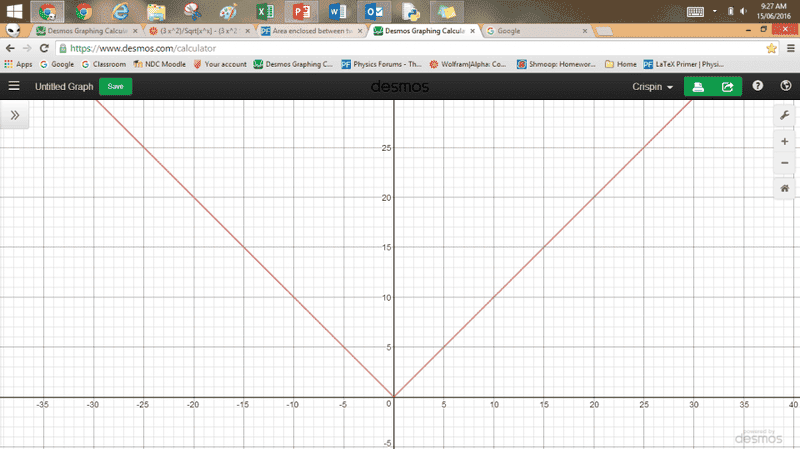# Area enclosed between two graphs

Saracen Rue

## Homework Statement

f(x) = √(x+2), g(x) = d/dx (f(x))^(f(x)). Find the total area enclosed between g(x) and √(x^2) correct to 3 decimal places.

## Homework Equations

Knowledge of differentiation and integration - specifically areas between curves.

## The Attempt at a Solution

I've attempted to solve g(x) = √(x^2) on my calculator but I keep receiving an error message. I've defined f(x) as equaling √(x+2), and I don't see where I could be going wrong. I know after I've found the points of intersection, all I need to do is set up definite integrals over the relevant domains with the graph on bottom over said domains being subtracted from the graph on top.

Homework Helper
Gold Member
I've attempted to solve g(x) = √(x^2) on my calculator but I keep receiving an error message.
It would be better to, instead of using a calculator, derive an explicit formula for g(x) by differentiating ##f(x)^{f(x)}##. It's a bit messy, but quite manageable. Then you can plot that function and it'll be fairly easy to see approximately where it must intersect ##\sqrt{x^2}##.

By the way, what is a simplified way to write ##\sqrt{x^2}##?

Saracen Rue
It would be better to, instead of using a calculator, derive an explicit formula for g(x) by differentiating ##f(x)^{f(x)}##. It's a bit messy, but quite manageable. Then you can plot that function and it'll be fairly easy to see approximately where it must intersect ##\sqrt{x^2}##.

By the way, what is a simplified way to write ##\sqrt{x^2}##?

Ah thank you for the advice. The square root of x squared simplifies to x, but the rule ##\sqrt{x^2}## sketches a distinctive graph due to the fact you must square x before square rooting it - this means y cannot be a negative number. Here's a picture to show you.Homework Helper
Gold Member
Good. Now try to differentiate ##\sqrt{(x+2)}^{\sqrt{x+2}}## to get a formula for the function ##g##.
It may help to first re-write it as ##e^{(x+2)^\frac12\cdot \frac12 \log(x+2)}##
You should get a function that is near zero at x=0 and asymptotically approaches zero from above as it heads left, and which then increases in a concave-up curve to the right. By roughly drawing this against the graph above you should be able to see how many times the two curves cross, and approximately where.

Mentor
Ah thank you for the advice. The square root of x squared simplifies to x, but the rule ##\sqrt{x^2}## sketches a distinctive graph due to the fact you must square x before square rooting it - this means y cannot be a negative number.
No, that's not why. ##\sqrt{x^2} = |x|##, the absolute value of x. If what you said was true -- that ##\sqrt{x^2}## simplifies to x, the graph would be a straight line through the origin, with a slope of 1.